# Articulos Matematicos

By ErickTV
• Period: Dec 27, 1571 to

## Johannes Kepler

Was a German astronomer, mathematician, and astrologer. He is a key figure in the 17th-century scientific revolution, best known for his laws of planetary motion, and his books Astronomia nova, Harmonices Mundi, and Epitome Astronomiae Copernicanae. These works also provided one of the foundations for Newton's theory of universal gravitation.
• Period: to

## René Descartes

Was a French-born philosopher, mathematician, and scientist who spent a large portion of his working life in the Dutch Republic, initially serving the Dutch States Army of Maurice of Nassau, Prince of Orange and the Stadtholder of the United Provinces. One of the most notable intellectual figures of the Dutch Golden Age,Descartes is also widely regarded as one of the founders of modern philosophy.
• # Mysterium CosmographicumIs an astronomy book by the German astronomer Johannes Kepler
• Period: to

## Pierre de Fermat

Was a French lawyer and a mathematician who is given credit for early developments that led to infinitesimal calculus, including his technique of adequality. He made notable contributions to analytic geometry, probability, and optics. He is best known for his Fermat's principle for light propagation and his Fermat's Last Theorem in number theory, which he described in a note at the margin of a copy of Diophantus' Arithmetica.
• # Mirifici Logarithmorum Canonis Descriptio.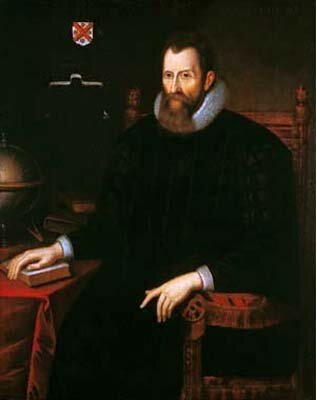John Napier discusses Napierian logarithms
• # La GéométrieThe work was the first to propose the idea of uniting algebra and geometry into a single subject and invented an algebraic geometry called analytic geometry, which involves reducing geometry to a form of arithmetic and algebra and translating geometric shapes into algebraic equations.
• # Harmonices Mundi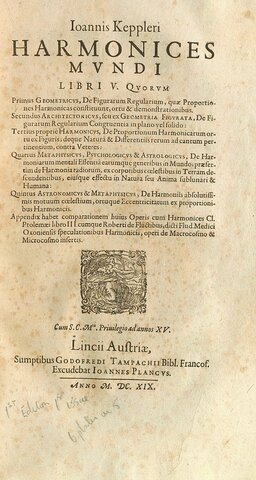Johannes Kepler discovers two of the Kepler-Poinsot polyhedra.
• Period: to

## Blaise Pascal

Was a French mathematician, physicist, inventor, philosopher, writer and Catholic theologian.
• # Fermat's Last Theorem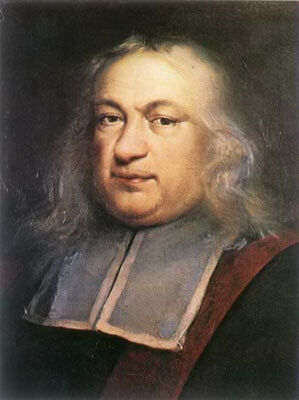Pierre de Fermat claims to have proven Fermat's Last Theorem in his copy of Diophantus' Arithmetica.
• Period: to

## Seki Takakazu

Was a Japanese mathematician and author of the Edo period. He has been described as "Japan's Newton".
He created a new algebraic notation system and, motivated by astronomical computations, did work on infinitesimal calculus and Diophantine equations. Although he was a contemporary of German polymath mathematician and philosopher Gottfried Leibniz and British mathematician Isaac Newton, Seki's work was independent.
• Period: to

## Sir Isaac Newton

Was an English mathematician, physicist, astronomer, theologian, and author ("natural philosopher") who is widely recognised as one of the greatest mathematicians and most influential scientists of all time and as a key figure in the scientific revolution. His book Philosophiæ Naturalis Principia Mathematica, established classical mechanics. Newton also made seminal contributions to optics, and shares credit with Gottfried Wilhelm Leibniz for developing the infinitesimal calculus.
• Period: to

## Gottfried Wilhelm (von) Leibniz

Was a prominent German polymath and one of the most important logicians, mathematicians and natural philosophers of the Enlightenment. As a representative of the seventeenth-century tradition of rationalism.
• # Traité du triangle arithmétique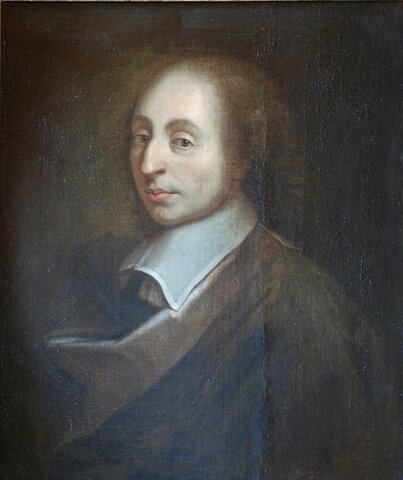Blaise Pascal and Pierre de Fermat create the theory of probability.
• # Kenpu no Hō1683 – Seki Takakazu discovers the resultant and determinant.
1683 – Seki Takakazu develops elimination theory.
• # De motu corporum in gyrumThis manuscript gave important mathematical derivations relating to the three relations now known as "Kepler's laws"
• # Nova Methodus pro Maximis et Minimis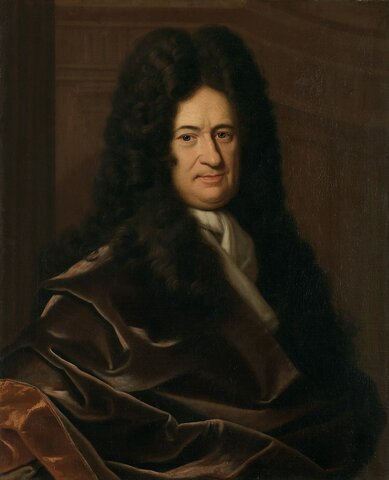iIs the first published work on the subject of calculus. It was published by Gottfried Leibniz. It is considered to be the birth of infinitesimal calculus.
• # Philosophiæ Naturalis Principia MathematicaThe Principia states Newton's laws of motion, forming the foundation of classical mechanics; Newton's law of universal gravitation; and a derivation of Johannes Kepler's laws of planetary motion, used mathematical methods now included in the field of calculus
• # Opticks: or, A Treatise of the Reflexions, Refractions, Inflexions and Colours of LightThe book analyzes the fundamental nature of light by means of the refraction of light with prisms and lenses, the diffraction of light by closely spaced sheets of glass, and the behaviour of color mixtures with spectral lights or pigment powders. It is considered one of the great works of science in history.
• Period: to

## Leonhard Euler

Was a Swiss mathematician, physicist, astronomer, geographer, logician and engineer who made important and influential discoveries in many branches of mathematics, such as infinitesimal calculus and graph theory, while also making pioneering contributions to several branches such as topology and analytic number theory. He is also known for his work in mechanics, fluid dynamics, optics, astronomy and music theory
• # Katsuyō Sanpō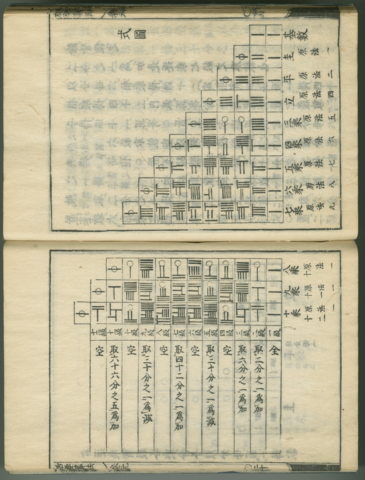Seki Takakazu discovers Bernoulli numbers. Jacob Bernoulli whom the numbers are named after is believed to have independently discovered the numbers shortly after Takakazu.
• Period: to

## Johann Heinrich Lambert

(German: [ˈlambɛʁt], Jean-Henri Lambert in French; 26 or 28 August 1728 – 25 September 1777) was a Swiss polymath who made important contributions to the subjects of mathematics, physics (particularly optics), philosophy, astronomy and map projections.
• # Mechanica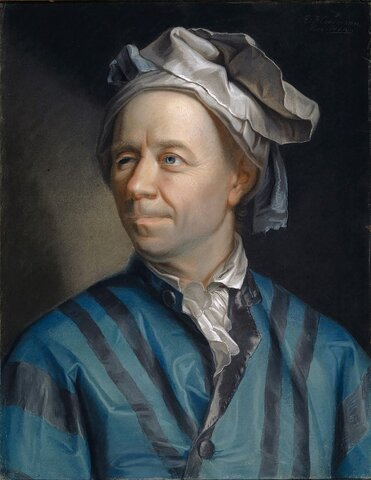Is a two-volume work published by mathematician Leonhard Euler which describes analytically the mathematics governing movement.Euler both developed the techniques of analysis and applied them to numerous problems in mechanics.
• Period: to

## Joseph-Louis Lagrange

Was an Italian mathematician and astronomer, later naturalized French. He made significant contributions to the fields of analysis, number theory, and both classical and celestial mechanics.
• # Goldbach's conjecture.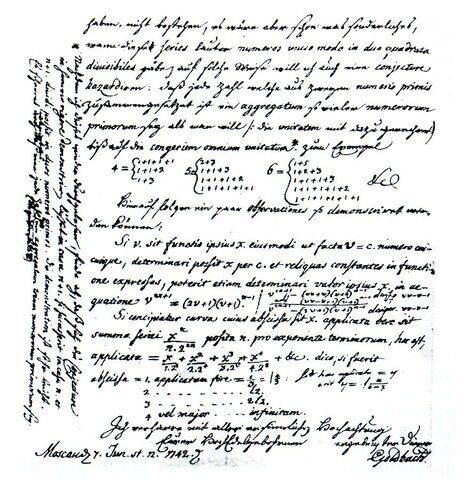Christian Goldbach conjectures that every even number greater than two can be expressed as the sum of two primes, now known as Goldbach's conjecture.
• # Methodus inveniendi lineas curvas maximi minimive proprietate gaudentes, sive Solutio problematis isoperimetrici latissimo sensu accepti.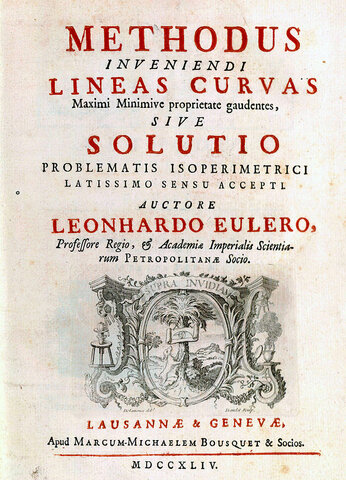Calculus of Variations
• # Introductio in analysin infinitorumIs a two-volume work by Leonhard Euler which lays the foundations of mathematical analysis
• Period: to

Was a French mathematician who made numerous contributions to mathematics. Well-known and important concepts such as the Legendre polynomials and Legendre transformation are named after him.
• # Beyträge zum Gebrauche der Mathematik und deren Anwendung II (1770)Johann Heinrich Lambert proves that π is irrational.
• Period: to

## Jean-Baptiste Joseph Fourier

Was a French mathematician and physicist born in Auxerre and best known for initiating the investigation of Fourier series, which eventually developed into Fourier analysis and harmonic analysis, and their applications to problems of heat transfer and vibrations. The Fourier transform and Fourier's law of conduction are also named in his honour. Fourier is also generally credited with the discovery of the greenhouse effect.
• Period: to

## Johann Carl Friedrich Gauss

Was a German mathematician and physicist who made significant contributions to many fields in mathematics and science. Sometimes referred to as the Princeps mathematicorum (Latin for '"the foremost of mathematicians"') and "the greatest mathematician since antiquity", Gauss had an exceptional influence in many fields of mathematics and science, and is ranked among history's most influential mathematicians.
• Period: to

## Augustin-Louis Cauchy

Was a French mathematician, engineer, and physicist who made pioneering contributions to several branches of mathematics, including mathematical analysis and continuum mechanics. He was one of the first to state and rigorously prove theorems of calculus, rejecting the heuristic principle of the generality of algebra of earlier authors. He almost singlehandedly founded complex analysis and the study of permutation groups in abstract algebra.
• Period: to

## Nikolai Ivanovich Lobachevsky

Was a Russian mathematician and geometer, known primarily for his work on hyperbolic geometry, otherwise known as Lobachevskian geometry and also his fundamental study on Dirichlet integrals known as Lobachevsky integral formula.
• # Theorie des fonctions analytiquesContenant les principes du calcul différentiel, dégagés de toute considération d'infiniment petits ou d'évanouissans, de limites ou de fluxions, et réduits a l'analyse algébrique des quantités finies. par J.L. Lagrange
• # Essai sur la théorie des nombresAdrien-Marie Legendre conjectures the prime number theorem.
• # New proof of the theorem that every integral algebraic function of one variable can be resolved into real factors (i.e., polynomials) of the first or second degree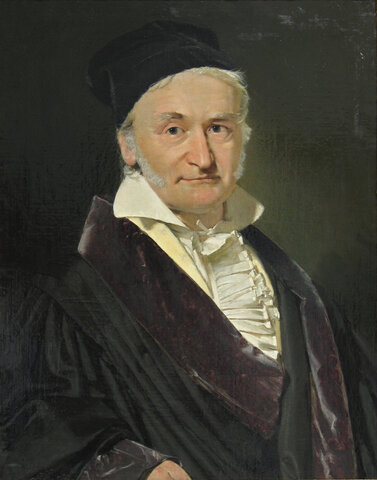Carl Friedrich Gauss proves the fundamental theorem of algebra (every polynomial equation has a solution among the complex numbers)
• # Disquisitiones Arithmeticae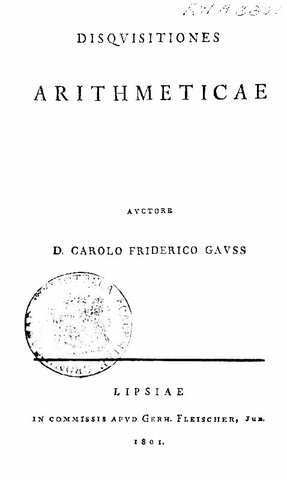Is a textbook of number theory written by Carl Friedrich Gauss in 1798 when Gauss was 21 and first published in 1801 when he was 24. It is notable for having had a revolutionary impact on the field of number theory as it not only made the field truly rigorous and systematic but also paved the path for modern number theory.
• Period: to

## Lejeune Dirichlet

Johann Peter Gustav Lejeune Dirichlet was a German mathematician who made deep contributions to number theory (including creating the field of analytic number theory), and to the theory of Fourier series and other topics in mathematical analysis; he is credited with being one of the first mathematicians to give the modern formal definition of a function.
• Period: to

## Sir William Rowan Hamilton

Was an Irish mathematician, worked inpure mathematics and mathematics for physics. He made important contributions to optics, classical mechanics and algebra. his work was of major importance to physics, particularly his reformulation of Newtonian mechanics, now called Hamiltonian mechanics. This work has proven central to the modern study of classical field theories such as electromagnetism, and quantum mechanics. In pure mathematics, he is best known as the inventor of quaternions.
• Period: to

## Évariste Galois

Matemático y activista político francés. Cuando aún era adolescente, pudo determinar una condición necesaria y suficiente para que un polinomio se pudiera resolver mediante radicales. Su trabajo sentó las bases de la teoría de Galois y la teoría de grupos. Murió a los 20 años por las heridas sufridas en un duelo.
• Period: to

## Karl Weierstrass

Karl Theodor Wilhelm Weierstrass was a German mathematician often cited as the "father of modern analysis". Despite leaving university without a degree, he studied mathematics and trained as a school teacher, eventually teaching mathematics, physics, botany and gymnastics.
• Period: to

## George Boole

Was a largely self-taught English mathematician, philosopher and logician, most of whose short career was spent as the first professor of mathematics at Queen's College, Cork in Ireland. He worked in the fields of differential equations and algebraic logic
• # Rein analytischer Beweis des Lehrsatzes, dass zwischen je zwey Werthen, die ein entgegengesetzes Resultat gewähren, wenigstens eine reele Wurzel der Gleichung liegeBernard Bolzano, presents the intermediate value theorem—a continuous function that is negative at one point and positive at another point must be zero for at least one point in between. Bolzano gives a first formal (ε, δ)-definition of limit.
• Period: to

## Sir George Gabriel Stokes

Was an Anglo-Irish physicist and mathematician, Stokes made seminal contributions to fluid mechanics, including the Navier–Stokes equations and to physical optics, with notable works on polarization and fluorescence. As a mathematician, he popularised "Stokes' theorem" in vector calculus and contributed to the theory of asymptotic expansions.
• # Cours d'Analyse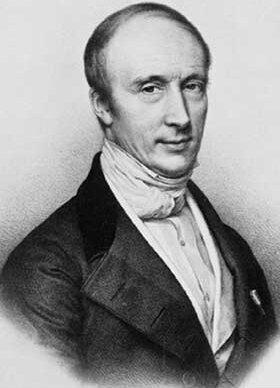• Period: to

## Arthur Cayley

Fue un matemático prolífico que trabajó en álgebra.
Postuló el teorema de Cayley-Hamilton: cada matriz cuadrada es una raíz de su propio polinomio característico , y lo verificó para matrices de orden 2 y 3. Fue el primero en definir el concepto de grupo en la forma moderna —Como un conjunto con una operación binaria que satisface ciertas leyes. Las tablas de Cayley y los gráficos de Cayley , así como el teorema de Cayley, se nombran en honor a Cayley.
• # The Analytical Theory of Heat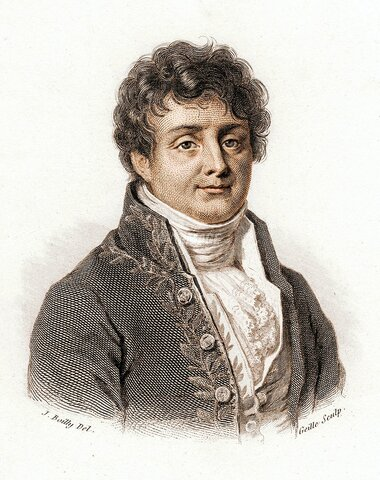Fourier claimed that any function of a variable, whether continuous or discontinuous, can be expanded in a series of sines of multiples of the variable.
• # Crelle's JournalNiels Henrik Abel partially proves the Abel–Ruffini theorem that the general quintic or higher equations cannot be solved by a general formula involving only arithmetical operations and roots.
• # Mémoire sur les intégrales définies, prises entre des limites imaginaires

Augustin-Louis Cauchy presents the Cauchy integral theorem for general integration paths—he assumes the function being integrated has a continuous derivative, and he introduces the theory of residues in complex analysis.
• Period: to

## Bernhard Riemann

Georg Friedrich Bernhard Riemann was a German mathematician who made contributions to analysis, number theory, and differential geometry. In real analysis, he is mostly known for the first rigorous formulation of the integral, the Riemann integral, and his work on Fourier series. His contributions to complex analysis include most notably the introduction of Riemann surfaces. His famous 1859 paper on the prime-counting function, containing the original statement of the Riemann hypothesis
• # On the Origin of Geometry (Lobachevsky)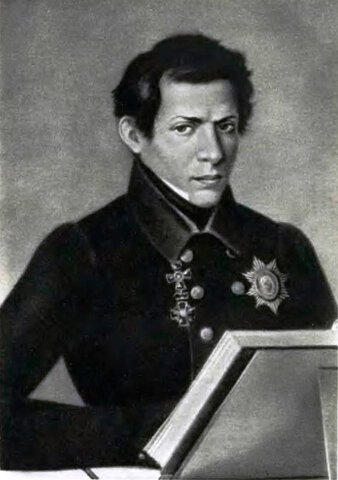János Bolyai, Gauss, and Lobachevsky invent hyperbolic non-Euclidean geometry.
• Period: to

## Richard Dedekind

Julius Wilhelm Richard Dedekind (6 October 1831 – 12 February 1916) was a German mathematician who made important contributions to abstract algebra (particularly ring theory), axiomatic foundation for the natural numbers, algebraic number theory and the definition of the real numbers.
• # Galois theory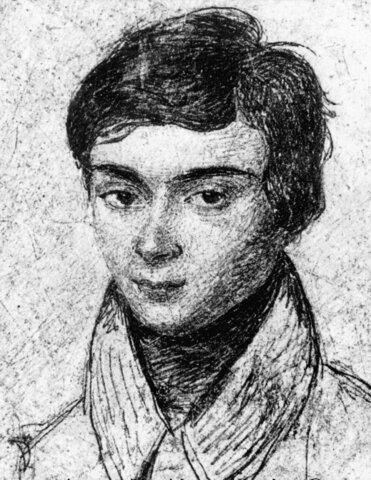Évariste Galois presents a general condition for the solvability of algebraic equations, thereby essentially founding group theory and Galois theory
• # Beweis des Satzes, dass jede unbegrenzte arithmetische Progression, deren erstes Glied und Differenz ganze Zahlen ohne gemeinschaftlichen Factor sind, unendlich viele Primzahlen enthält.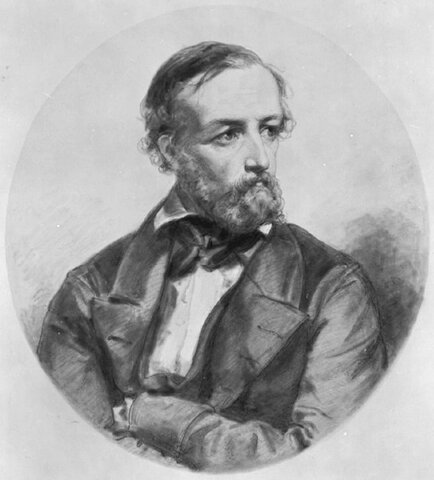Dirichlet: Analytic number theory.
• Period: to

## Marius Sophus Lie

Was a Norwegian mathematician. He largely created the theory of continuous symmetry and applied it to the study of geometry and differential equations.
• Period: to

## Georg Ferdinand Ludwig Philipp Cantor

Was a German mathematician. He created set theory, which has become a fundamental theory in mathematics. Cantor established the importance of one-to-one correspondence between the members of two sets, defined infinite and well-ordered sets, and proved that the real numbers are more numerous than the natural numbers. In fact, Cantor's method of proof of this theorem implies the existence of an infinity of infinities. He defined the cardinal and ordinal numbers and their arithmetic.
• # Vandenhoeck und RuprechtJohann Benedict Listing (25 July 1808 – 24 December 1882) was a German mathematician. He first introduced the term "topology", in a famous article published in 1847
• # The Mathematical Analysis of Logic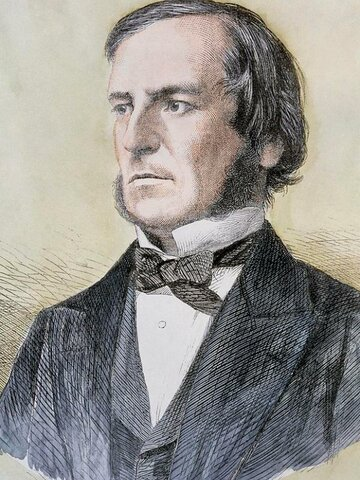George Boole formalizes symbolic logic in The Mathematical Analysis of Logic, defining what is now called Boolean algebra.
• # Beitrag zur Theorie der Abel'schen IntegraleKarl Weierstrasse "Las relaciones que he desarrollado son
de particular importancia para la teoría de los trascendentes abelianos."
• # "On the dynamical theory of diffraction"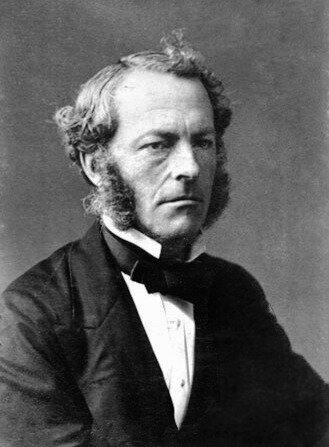George Gabriel Stokes shows that solitary waves can arise from a combination of periodic waves.
• Period: to

## Christian Felix Klein

Was a German mathematician and mathematics educator, known for his work with group theory, complex analysis, non-Euclidean geometry, and on the associations between geometry and group theory. His 1872 Erlangen program, classifying geometries by their basic symmetry groups, was an influential synthesis of much of the mathematics of the time.
• # "On the numerical calculation of a class of definite integrals and infinite series,"

George Gabriel Stokes rediscovers and proves Stokes' theorem.
• # Lectures on QuaternionsWilliam Hamilton discovers the calculus of quaternions and deduces that they are non-commutative. Lectures on quaternions : containing a systematic statement of a new mathematical method, of which the principles were communicated in 1843
• # "On the theory of groups, as depending on the symbolic equation θn = 1,"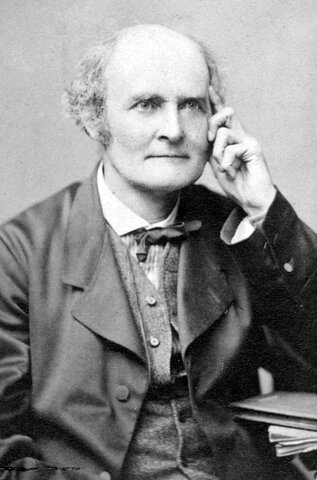Arthur Cayley shows that quaternions can be used to represent rotations in four-dimensional space
• # On the hypotheses which underlie geometry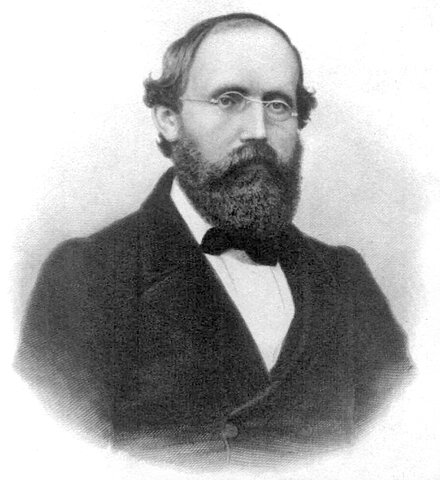Bernhard Riemann introduces Riemannian geometry.
• # An Investigation of the Laws of Thought

Is the second of Boole's two monographs on algebraic logic.
• Period: to

## Jules Henri Poincaré

Was a French mathematician, theoretical physicist, engineer, and philosopher of science. He is often described as a polymath, and in mathematics as "The Last Universalist"
• # Möbius strip.August Ferdinand Möbius invents the Möbius strip.
• # On the Number of Primes Less Than a Given MagnitudeBernhard Riemann formulates the Riemann hypothesis, which has strong implications about the distribution of prime numbers.
• Period: to

## David Hilbert

Was a German mathematician and one of the most influential mathematicians of the 19th and early 20th centuries. Hilbert discovered and developed a broad range of fundamental ideas in many areas, including invariant theory, the calculus of variations, commutative algebra, algebraic number theory, the foundations of geometry, spectral theory of operators and its application to integral equations, mathematical physics, and the foundations of mathematics (particularly proof theory).
• # Stetigkeit und irrationale Zahlen (Dedekind)Richard Dedekind invents what is now called the Dedekind Cut for defining irrational numbers, and now used for defining surreal numbers.
• # Vergleichende Betrachtungen über neuere geometrische ForschungenErlangen program is a method of characterizing geometries based on group theory and projective geometry. It was published by Felix Klein
• # On a Property of the Collection of All Real Algebraic Numbers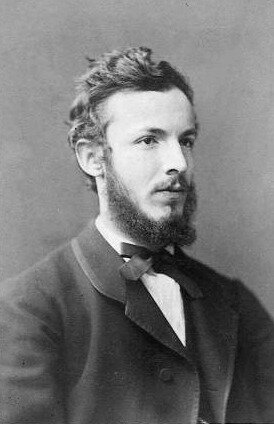Georg Cantor proves that the set of all real numbers is uncountably infinite but the set of all real algebraic numbers is countably infinite.
• # Klein bottleFelix Klein invents the Klein bottle.
• Period: to

## Amalie Emmy Noether

Was a German mathematician who made many important contributions to abstract algebra. She discovered Noether's theorem, which is fundamental in mathematical physics. She developed some theories of rings, fields, and algebras. In physics, Noether's theorem explains the connection between symmetry and conservation laws.
• # Theorie der Transformationsgruppen ILie's principal tool, and one of his greatest achievements, was the discovery that continuous transformation groups (now called, after him, Lie groups) could be better understood by "linearizing" them, and studying the corresponding generating vector fields (the so-called infinitesimal generators). The generators are subject to a linearized version of the group law, now called the commutator bracket, and have the structure of what is today called a Lie algebra
• # Was sind und was sollen die Zahlen? (Richard Dedekind)

Richard Dedekind (1888).
• # ANALYSIS SITUS (Poincaré)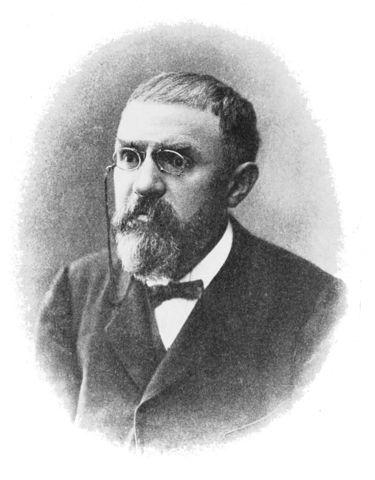The first systematic study of topology
• # Beiträge zur Begründung der transfiniten Mengenlehre

Georg Cantor publishes a book about set theory containing the arithmetic of infinite cardinal numbers and the continuum hypothesis.
• # Grundlagen der GeometrieDavid Hilbert presents a set of self-consistent geometric axioms in Foundations of Geometry
• # Cantor discovers a contradiction in his set theory

Georg Cantor discovers a contradiction in his set theory
• # Hilbert Problems

David Hilbert states his list of 23 problems, which show where some further mathematical work is needed.
• Period: to

## Kurt Friedrich Gödel

Was a Austrian-German-American logician, mathematician, and analytic philosopher. Considered along with Aristotle and Gottlob Frege to be one of the most significant logicians in history, Gödel had an immense effect upon scientific and philosophical thinking in the 20th century, a time when others such as Bertrand Russell, Alfred North Whitehead, and David Hilbert were analyzing the use of logic and set theory to understand the foundations of mathematics pioneered by Georg Cantor.
• # Der Endlichkeitssatz der Invarianten endlicher Gruppen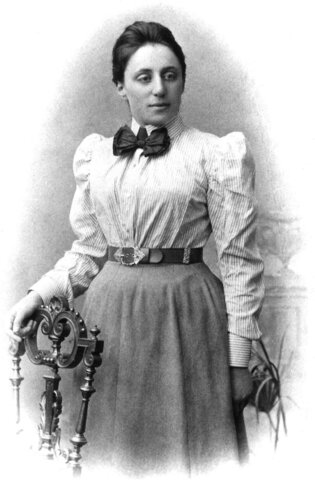Emmy Noether proves her symmetry theorem, which shows that every symmetry in physics has a corresponding conservation law
• # Theory of Ideals in Ring Domains

Emmy Noether introduces the first general definition of a commutative ring.
• Period: to

## Alexander Grothendieck

His research extended the scope of the field and added elements of commutative algebra, homological algebra, sheaf theory and category theory to its foundations, while his so-called "relative" perspective led to revolutionary advances in many areas of pure mathematics.
• # Hypercomplex Quantities and the Theory of Representations

Emmy Noether introduces the first general representation theory of groups and algebras
• # On formally undecidable propositions of Principia Mathematica and related systemsKurt Gödel proves his incompleteness theorem, which shows that every axiomatic system for mathematics is either incomplete or inconsistent
• # The Consistency of the Axiom of Choice and of the Generalized Continuum Hypothesis with the Axioms of Set Theory

Kurt Gödel shows that neither the continuum hypothesis nor the axiom of choice can be disproven from the standard axioms of set theory.
• # Produits tensoriels topologiques et espaces nucléaires• # "On manifolds homeomorphic to the 7-sphere"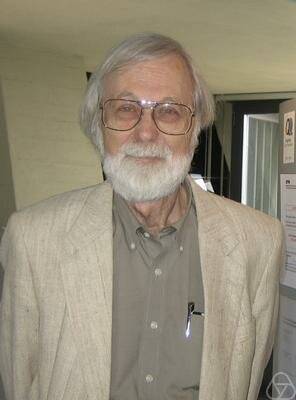John Milnor discovers the existence of an Exotic sphere in seven dimensions, inaugurating the field of differential topology.

• # "Le théorème de Riemann-Roch (d'après des résultats inédits de A. Grothendieck)",

Alexander Grothendieck's proof of the Grothendieck–Riemann–Roch theorem is published

• # "The independence of the continuum hypothesis"Paul Cohen uses his technique of forcing to show that neither the continuum hypothesis nor the axiom of choice can be proven from the standard axioms of set theory.
• # Euler Products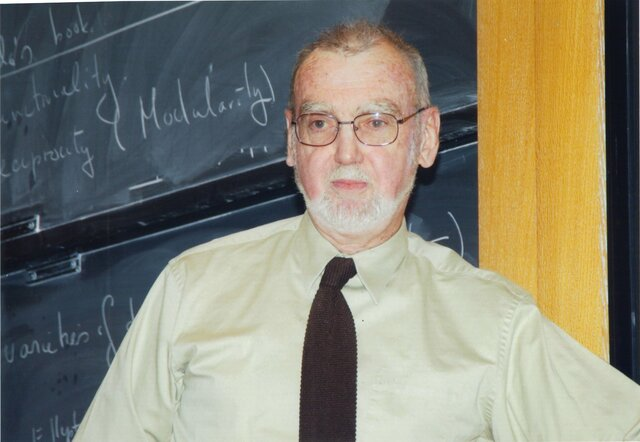Robert Langlands formulates the influential Langlands program of conjectures relating number theory and representation theory
• # The Index of Elliptic OperatorsMichael Atiyah and Isadore Singer prove the Atiyah–Singer index theorem about the index of elliptic operators.
• # "La conjecture de Weil. I"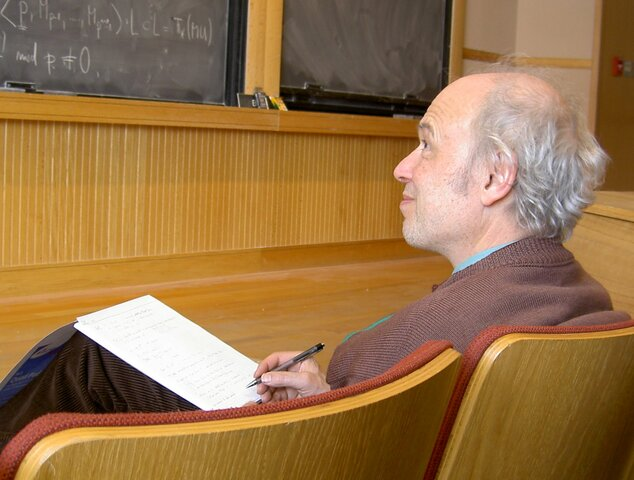Pierre Deligne solves the last and deepest of the Weil conjectures, completing the program of Grothendieck.
• # Fractals: Form, Chance and Dimension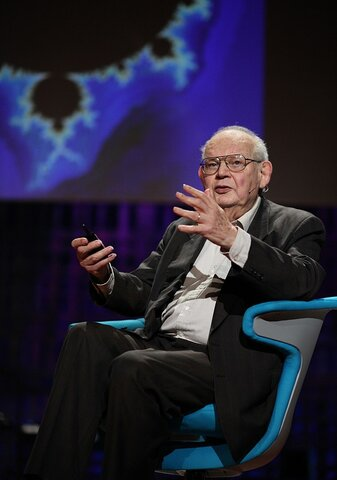Benoît Mandelbrot publishes Les objets fractals, forme, hasard et dimension
• # "Three-dimensional manifolds, Kleinian groups and hyperbolic geometry"Thurston's geometrization conjecture states that each of certain three-dimensional topological spaces has a unique geometric structure that can be associated with it. It is an analogue of the uniformization theorem for two-dimensional surfaces, which states that every simply connected Riemann surface can be given one of three geometries (Euclidean, spherical, or hyperbolic).
• # Modular elliptic curves and Fermat's Last Theorem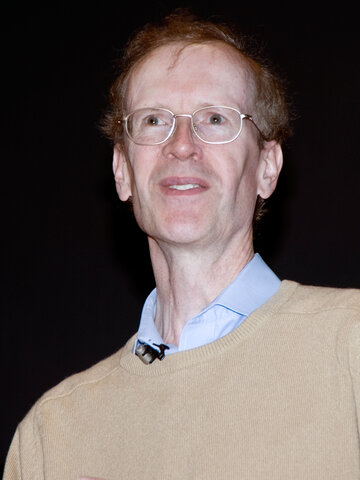Andrew Wiles proves part of the Taniyama–Shimura conjecture and thereby proves Fermat's Last Theorem
• # Millennium Prize Problems

The Clay Mathematics Institute proposes the seven Millennium Prize Problems of unsolved important classic mathematical questions
• # Ricci flow with surgery on three-manifolds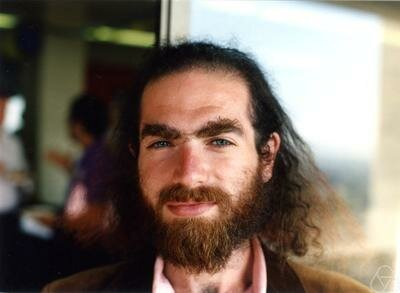Grigori Perelman proves the Poincaré conjecture.